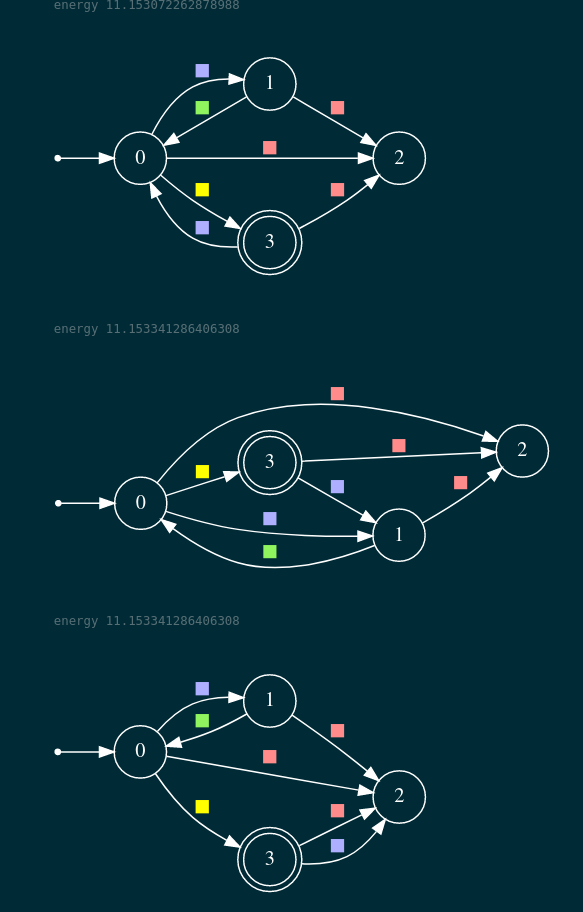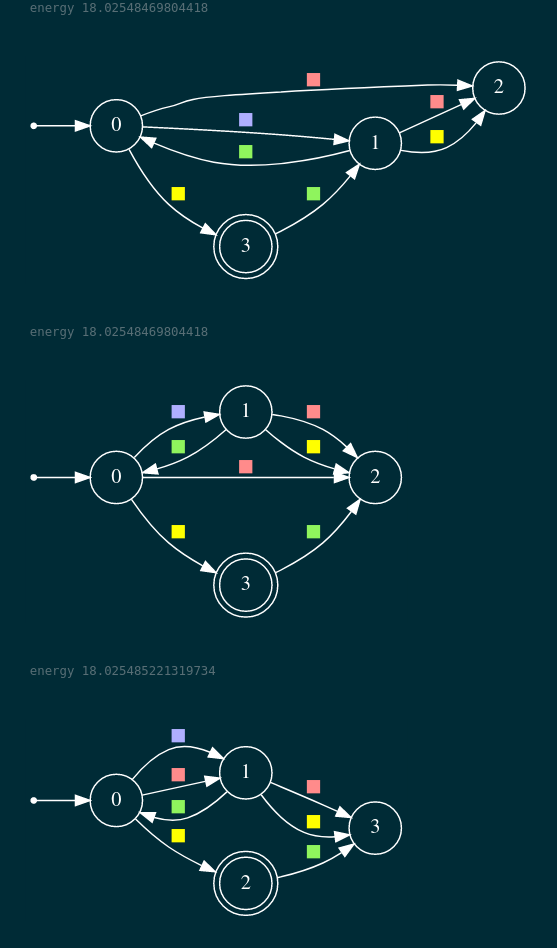# Demonstration Informed Specification Search

## A Maximum Entropy Approach

### Marcell Vazquez-Chanlatte

#### PhD Candidate at UC Berkeley

Joint work with Ameesh Shah, Gil Lederman, and Sanjit A. Seshia

# Structure of the talk

## Specification motivated agents## Likelihood Calculation in Symbolic MDPs

"Learning Task Specifications from Demonstrations." NeurIPS 18.
"Maximum Causal Entropy Specification Inference from Demonstrations." CAV 20
"Entropy Guided Control Improvisation", RSS 21

# Structure of the talk

## Specification motivated agents## Likelihood Calculation in Symbolic MDPs

"Learning Task Specifications from Demonstrations." NeurIPS 18.
"Demonstration Informed Specification Search." In progress.

# Structure of the talk

## Specification motivated agents# Structure of the talk

## Specification motivated agents## Likelihood Calculation in Symbolic MDPs

"A Model Counter's Guide to Probabilistic Systems.". (preprint)
"Model Checking Finite-Horizon Markov Chains with Probabilistic Inference", CAV 21
"Maximum Causal Entropy Specification Inference from Demonstrations." CAV 20
"Entropy Guided Control Improvisation", RSS 21

# Many important domains benefit from goal inference.# Partial Communication through actions

1. Legible actions can be incredibly diagnostic.
Dragan, et al, "Legibility and predictability of robot motion." HRI 13.
2. Actions influence other agents.
Sadigh, et al. "Planning for autonomous cars that leverage effects on human actions." RSS 16.
3. Essential for interpreting other signals: (Language, Disengagments, Other agents)
Goodman, et al. "Pragmatic language interpretation as probabilistic inference." TiCS 16
McPherson, et al. "Modeling supervisor safe sets for improving collaboration in human-robot teams." IROS 18
Afolabi, et al. "People as sensors: Imputing maps from human actions." IROS 18.

# Consider an agent acting in the following stochastic grid world.# Can try to move up, down, left, right# May slip due to wind# What is the agent trying to do?# Probably trying to reach yellow tiles# Although these actions are surprising under that hypothesis# And isn't it easier to go to this yellow tile any way?# Goal: Systemize analysis to learn tasks# Demonstration Informed Specification Search

## D.I.S.S.# Will explore inferring goals as Boolean specifications

1. Support composition.
3. Be semantically robust to changes in the dynamics.

# Support boolean compositions# Support incremental learning# Don't need "wet" to describe dynamics# Can learn as state machine over state colors# Monolithic description# Easy to express variations# Contributions

1. Efficently learn finite trace properties from unlabeled and incomplete demonstrations in a Markov Decison Process.
2. Support incremental and monolithic learning.
3. Only needs blackbox access to a MaxEnt planner and Concept Identifer.

## Specification motivated agents## Conclusion and future work

"Learning Task Specifications from Demonstrations." NeurIPS 18.
"Maximum Causal Entropy Specification Inference from Demonstrations." CAV 20
"Entropy Guided Control Improvisation", RSS 21

# Dynamics Model

1. Assume some fixed sets of states and actions.2. A trace, $\xi$, is a sequence of states and actions.
3. Assume maximum episode length of, $$\tau \in \mathbb{N}$$.

# Trace properties

1. A (Boolean) specification, $\varphi$, is a set of traces.2. We say $\xi$ satisfies $\varphi$, if $\xi \in \varphi$.
3. A demonstration of a task $\varphi$ is a possibly unlabeled and incomplete example where the agent tries to satisfy $\varphi$.

# Trace properties derived from Formal Logic, Automata, etcWill call a collection of trace properties a concept class.# No a-priori order on traces# Agent model induces ordering.

1. Need to know what moves are "risky".
2. Need to know agent's objective and competency.# Frame as Inverse Reinforcement Learning

$\max_{\pi} \Big(\mathbb{E}_{s_{1:\tau}}\big(\sum_{i=1}^\tau r(s_i)~|~\pi\big)\Big)$
where $\pi(a~|~s) = \Pr(a~|~s)$

Given a series of demonstrations, what reward, $r(s)$, best explains the behavior? (Abbeel and Ng 2004)

# No unique explanatory reward!

$\max_{\pi} \Big(\mathbb{E}_{s_{1:\tau}}\big(\sum_{i=1}^\tau r(s_i)~|~\pi\big)\Big)$

## Maximize Causal Entropy

$H(A_{1:\tau}~||~S_{1:\tau}) = \sum_{t=1}^T H\Big(A_{t} \mid S_{1:t}\Big)$

subject to feature statistics.

(Ziebart, et al. 2010)

# Focus on reward matching

$\max_{\pi} \Big(\mathbb{E}_{s_{1:\tau}}\big(\sum_{i=1}^\tau r(s_i)~|~\pi\big)\Big)$

## Maximize Causal Entropy

$H(A_{1:\tau}~||~S_{1:\tau}) = \sum_{t=1}^T H\Big(A_{t} \mid S_{1:t}\Big)$

subject to $E[r]$

# What should the reward be?## Proposal: Use indicator.

$r(\xi) \triangleq \begin{cases} 1 & \text{if } \xi \in \varphi\\ 0 & \text{otherwise} \end{cases}$

$E[r] = \Pr(\xi \in \varphi)$

# Maximum Causal Entropy Policy

\begin{equation} \log\big(\pi_{\mathbf{\lambda}}(a~|~s_{1:t})\big) = Q_{\mathbf{\lambda}}(s_{1:t}, a) - V_{\mathbf{\lambda}}(s_{1:t}) \end{equation}
where
$V_{\mathbf{\lambda}}(s_{1:t}) \triangleq \begin{cases} \ln \sum_{a} e^{Q_{\mathbf{\lambda}}(s_{1:t}, a)} & \text{if } t \neq \tau,\\ \lambda\cdot [s_{1:\tau} \in \varphi] & \text{otherwise}. \end{cases}$
$Q_{\mathbf{\lambda}}(s_{1:t}, a) \triangleq \mathbb{E}_{s_{1:t+1}}\left[ V_{\mathbf{\lambda}}(s_{t+1})~|~s_{1:t}, a\right]$

Find $\lambda$ to match $p^*$.

# Entropy regularized bellman backup

\begin{equation} \log\big(\pi_{\mathbf{\lambda}}(a~|~s_{1:t})\big) = Q_{\mathbf{\lambda}}(s_{1:t}, a) - V_{\mathbf{\lambda}}(s_{1:t}) \end{equation}
where
$V_{\mathbf{\lambda}}(s_{1:t}) \triangleq \begin{cases} \ln \sum_{a} e^{Q_{\mathbf{\lambda}}(s_{1:t}, a)} & \text{if } t \neq \tau,\\ \lambda\cdot [s_{1:\tau} \in \varphi] & \text{otherwise}. \end{cases}$
$Q_{\mathbf{\lambda}}(s_{1:t}, a) \triangleq \mathbb{E}_{s_{1:t+1}}\left[ V_{\mathbf{\lambda}}(s_{t+1})~|~s_{1:t}, a\right]$

max ↦ log sum exp.

$\mathbb{E}[r(s_{1:t})] \mapsto \mathbb{E}[r(s_{1:t})] + H(a\mid s_{1:t})$
(Geist et al, ICML '19)

# Entropy regularized bellman backup

\begin{equation} \log\big(\pi_{\mathbf{\lambda}}(a~|~s_{1:t})\big) = Q_{\mathbf{\lambda}}(s_{1:t}, a) - V_{\mathbf{\lambda}}(s_{1:t}) \end{equation}
where
$V_{\mathbf{\lambda}}(s_{1:t}) \triangleq \begin{cases} \text{smax}_{a} Q(s_{1:t}, a) & \text{if } t \neq \tau,\\ \lambda\cdot [s_{1:\tau} \in \varphi] & \text{otherwise}. \end{cases}$
$Q_{\mathbf{\lambda}}(s_{1:t}, a) \triangleq \mathbb{E}_{s_{1:t+1}}\left[ V_{\mathbf{\lambda}}(s_{t+1})~|~s_{1:t}, a\right]$

max ↦ smooth max (smax).

# Take as blackbox for now

\begin{equation} \log\big(\pi_{\mathbf{\lambda}}(a~|~s_{1:t})\big) = Q_{\mathbf{\lambda}}(s_{1:t}, a) - V_{\mathbf{\lambda}}(s_{1:t}) \end{equation}
where
$V_{\mathbf{\lambda}}(s_{1:t}) \triangleq \begin{cases} \text{smax}_{a} Q(s_{1:t}, a) & \text{if } t \neq \tau,\\ \lambda\cdot [s_{1:\tau} \in \varphi] & \text{otherwise}. \end{cases}$
$Q_{\mathbf{\lambda}}(s_{1:t}, a) \triangleq \mathbb{E}_{s_{1:t+1}}\left[ V_{\mathbf{\lambda}}(s_{t+1})~|~s_{1:t}, a\right]$## Specification motivated agents# Demonstration Informed Specification Search# Focus on surprise guided sampler# Goal

Want to find specification that makes the demonstrations unsurprising.

$h(\varphi) \triangleq -\sum_{i=1}^n \ln \Pr(\text{demo}_i~|~\varphi, \text{dynamics})$

# Three key ideas

Want to find specification that makes the demonstrations unsurprising.

$h(\varphi) \triangleq -\sum_{i=1}^n \ln \Pr(\text{demo}_i~|~\varphi, \text{dynamics})$
1. Reframe problem as conforming or deviating from demonstrations.
2. View demonstration prefix tree as computation graph.
3. Can manipulate value of subtree by toggling trace labels.

# Many ways to deviate from demonstration

Each deviation's subtree summarized by $V_\lambda, Q_\lambda$.

# Reframe as conforming or deviating

$\mathbb{E}$ and $\text{smax}$ are associative

$\begin{split} \text{smax}(x, y, z) &= \ln(e^x + e^y + e^z)\\ &= \ln(e^x + e^{\ln(e^y + e^z)})\\ &= \text{smax}(x, \text{smax}(y, z)) \end{split}$

So we can summarize value of "pivoting" at a given node.

# Surprise factors through a function over pivot values.

$\widehat{h} : \mathbb{R}^{d} \to [0, 1]$
$h(\varphi) = \widehat{h}(\vec{V}_\varphi)$

# Gradient of proxy surprise easy to compute.

$\frac{\partial \widehat{h}}{\partial V_k}(\vec{V}) = \sum_{\substack{(i, j) \in E\\i \text{ is ego}}} \#_{(i, j)} \cdot \bigg(\Pr(i \rightsquigarrow k\mid \vec{V}) - \Pr(j \rightsquigarrow k\mid \vec{V})\bigg)$

Key idea 3: Toggle trace labels to manipulate pivot values.

# Flipping value monotonically changes subtree value

$V_3 < V_3'$

Can sample from $\pi$ to generate high weight paths.

# Surprise Guided Sampler

1. Fix a candidate spec and compute proxy gradient.
2. Define the pivot distribution, $D$ = softmax $\left (\frac{|\nabla \widehat{h}|}{\beta}\right)$.
3. Sample a pivot, $k \sim D$ and a path, $\xi$, using the $\pi_\varphi$ such that:
1. $\xi$ pivots at $k$.
2. $\nabla_k \widehat{h} > 0 \iff \xi \in \varphi$.
4. New label given by $\nabla_k \widehat{h} < 0$.# Candidate sampler can be any exact learner# Lifted in simulated annealing using example buffer# Demonstration Informed Specification Search# What is the agent trying to do?# Quickly finds high mass probability region# Top 3 DFAs found# Can handle monolithic synthesis as well# DISS able to find high mass probability region# Top 3 DFAs found# In this talk

1. Max (causal) entropy forecasting of specification driven agents in MDPs and Stochastic Games
"Learning Task Specifications from Demonstrations." NeurIPS 18.
"Maximum Causal Entropy Specification Inference from Demonstrations." CAV 20
"Entropy Guided Control Improvisation", RSS 21
2. Searching for likely specifications given demonstrations
"Learning Task Specifications from Demonstrations." NeurIPS 18.
"Demonstration Informed Specification Search." In progress.

# By no means solved# Clear path to scaling up

1. Need a way to estimate values.
2. Need a way to sample likely paths from policy.

# Plays nicely with approximate methods

1. Dynamic Programming
2. Dynamic Programming on compressed structure
3. Monte Carlo Tree Search (e.g., Smooth Cruiser )
4. Function Approximation (e.g., Soft Actor Critic )
 Grill, et al. "Planning in entropy-regularized Markov decision processes and games." NeurIPs19.
 Haarnoja, et al. "Soft actor-critic: Off-policy maximum entropy deep reinforcement learning with a stochastic actor." PMLR, 18.

# Natural path for combining symbolic and MCTS methods

1. Dynamic Programming
2. Dynamic Programming on compressed structure
3. Monte Carlo Tree Search (e.g., Smooth Cruiser )
4. Function Approximation (e.g., Soft Actor Critic )
 Grill, et al. "Planning in entropy-regularized Markov decision processes and games." NeurIPs19.
 Haarnoja, et al. "Soft actor-critic: Off-policy maximum entropy deep reinforcement learning with a stochastic actor." PMLR, 18.

# Multi-agent (inferred) assume guarantee reasoning underexplored

$A \implies G$Maximum causal entropy correlated equillibria seem like an interesting model
Ziebart, et al., "Maximum causal entropy correlated equilibria for Markov games." AAAI 10.